# Core

Model of core losses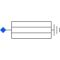# Information

This information is part of the Modelica Standard Library maintained by the Modelica Association.

Core losses can be separated into eddy current and hysteresis losses. The total core losses can thus be expressed as

```  P = PRef * (ratioHysteresis * (wRef / w) + 1 - ratioHysteresis) * (V / VRef)^2
```

where `w` is the actual angular remagnetization velocity and `V` is the actual voltage. The term `ratioHysteresis` is the ratio of the hysteresis losses with respect to the total core losses for the reference voltage and frequency.

In the current implementation hysteresis losses are not considered since complex numbers are not provided in Modelica. Therefore, implicitly `ratioHysteresis = 0` is set. For the voltage and frequency range with respect to Fig. 1, the dependency of total core losses on the parameter `ratioHysteresis` is depicted in Fig. 2. The current implementation has thus the drawback over a model that considers `ratioHysteresis > 0`:

• underestimation of the losses in the constant field region (`w` < `wRef`)
• overestimation of the losses in the field weakening region (`w` > `wRef`)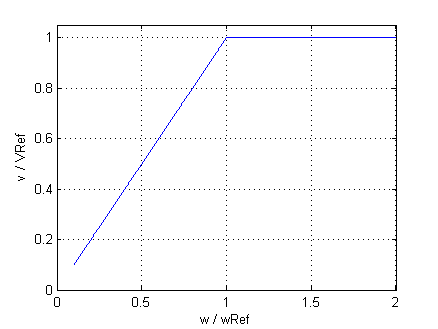Fig. 1: Voltage versus angular velocity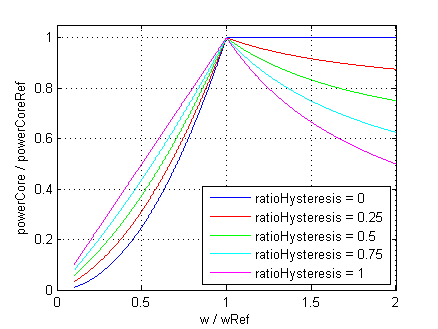Fig. 2: Core losses versus angular velocity with parameter `ratioHysteresis`

#### Note

In the current implementation it is assumed that `ratioHysteresis = 0`. This parameter cannot be changed due to compatibility reasons.

# Parameters (3)

useHeatPort Value: false Type: Boolean Description: =true, if heatPort is enabled Value: Type: CoreParameters Value: Type: Real Description: Effective number of stator turns / effective number of rotor turns (if used as rotor core)

# Inputs (1)

w Type: AngularVelocity (rad/s) Description: Remagnetization angular velocity

# Connectors (2)

heatPort spacePhasor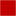Type: HeatPort_a Description: Optional port to which dissipated losses are transported in form of heat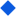Type: SpacePhasor

# Components (1)

 coreParameters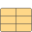Type: CoreParameters

# Used in Components (2)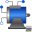AIM_SlipRing Modelica.Electrical.Machines.BasicMachines.AsynchronousInductionMachines Asynchronous induction machine with slipring rotor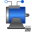PartialBasicInductionMachine Modelica.Electrical.Machines.Interfaces Partial model for induction machine